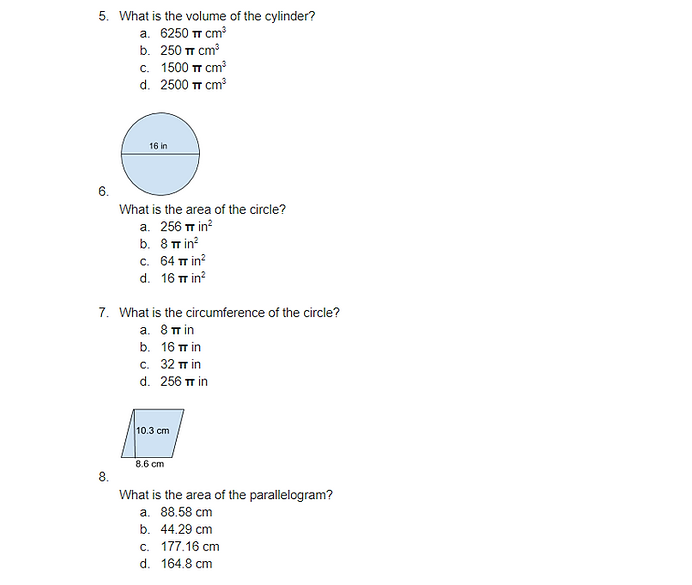top of pageGeometry

Welcome to the guide on Shapes, Area, and Volume

Before you start: This is a longer lesson so feel free to skip over concepts you are already comfortable with

Basic concepts of shapes:

https://youtu.be/E_-3ulbtcLk (Properties of polygons)

https://youtu.be/CA5O69Fv9NY (Perimeter)

https://youtu.be/1k0G-Y41jRA (Classifying triangles)

Note: Try to watch this video to get a better understanding of apothems, uses basic trigonometry

REMEMBER: Regular polygons are equiangular and equilateral, meaning all their sides are the same length and all their angles are the same degree.

Area:

https://www.youtube.com/watch?v=OC2rMWzc-I8 (Area of a square using diagonals)

Note: Uses trigonometry

Volume and Surface Area:

https://www.mathsisfun.com/geometry/sphere-volume-area.html (Spheres-Formulas)

https://www.mathsisfun.com/geometry/cuboids-rectangular-prisms.html (Volume and surface area for cuboids, rectangular prisms, and cubes)

Note: How can you modify the formula to only fit cubes? Remember, all the sides of a cube are the same length.

https://youtu.be/aEpkAtf2L54 (Cylinders-Video)

https://youtu.be/JN3LhO0YXw8 (Cones-Video)

Practice:

https://www.khanacademy.org/math/basic-geo/basic-geo-area-and-perimeter/quiz/basic-geo-area-and-perimeter-quiz-1?modal=1 (Area and perimeter of rectangles)

https://www.ixl.com/math/geometry/surface-area-and-volume-review (Surface area and volume)

https://www.ixl.com/math/grade-7/surface-area-of-cubes-and-prisms (Surface area of cubes and prisms)

https://www.ixl.com/math/grade-7/volume-of-cubes-and-prisms (Volumes of cubes and prisms)

https://cdn.kutasoftware.com/Worksheets/Geo/10-Surface%20Area%20of%20Prisms%20and%20Cylinders.pdf (Surface area of prisms and cylinders)

https://www.math-drills.com/measurement/cones_volume_surfacearea_whole_001.pdf?v=1427309164 (Surface area and volume of cones)

https://cdn.kutasoftware.com/Worksheets/Geo/10-Spheres.pdf (Surface area and volume of spheres)

https://cdn.kutasoftware.com/Worksheets/Geo/10-Volume%20of%20Pyramids%20and%20Cones.pdf (Volume of pyramids and cones)

_____________________________________________________________________________________________________

Check for Understanding (final review):